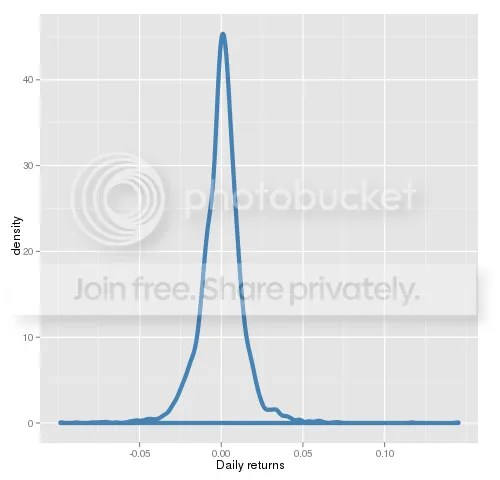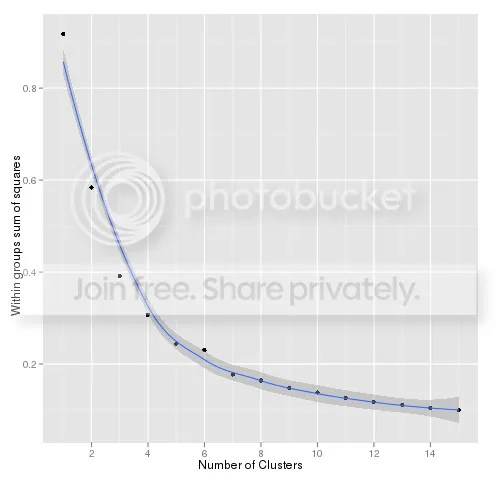Want to share your content on R-bloggers? click here if you have a blog, or here if you don't.

There is many flavors of artificial intelligence (AI), however I want to show practical example of the cluster analysis. It is very applicable in finance. For example, one of stylized facts of volatility is, that it moves in clusters, meaning that today’s volatility will be more likely as yesterday’s volatility. To gauge these moves you can use hidden Markov chain (complicated method) or k-means (probably to simplified). However, GARCH model successfully exploits this stylized fact to make prediction of tomorrow’s volatility (it takes into account another fact as well – volatility is mean reverting process).

K-means is based on unsupervised learning – you give the data and k-means decides how to classify it. The idea is to split data into clusters based on cluster center and assign each point to nearest center.  There is drawback with such approach – the algorithm tries to establish the centers of  clusters with initial data set. If the data is very noisy and the centers are not stable, then every try will give you different results.

As you probably know, the distribution of financial data is very unstable. How to tackle this problem? We should be looking at daily returns instead of prices. The figure below shows daily returns of SPY stock.

?View Code RSPLUS
 ```setwd('/home/git/Rproject/kmeans/') require(quantmod) require(ggplot2) Sys.setenv(TZ="GMT") getSymbols('SPY',from='2000-01-01')   x=data.frame(d=index(Cl(SPY)),return=as.numeric(Delt(Cl(SPY)))) png('daily_density.png',width=500) ggplot(x,aes(return))+stat_density(colour="steelblue", size=2, fill=NA)+xlab(label='Daily returns') dev.off()```I was ready to show another trick – how to neutralize long tails by replacing existing distribution with uniform distribution, but quick test revealed, that this leads to uninterpretable results.

OK, lets move further – how many clusters should we have? Can AI give us a clue? Of course, but keep in mind that then your future decision will be anchored.

?View Code RSPLUS
 ```nasa=tail(cbind(Delt(Op(SPY),Hi(SPY)),Delt(Op(SPY),Lo(SPY)),Delt(Op(SPY),Cl(SPY))),-1)   #optimal number of clusters wss = (nrow(nasa)-1)*sum(apply(nasa,2,var)) for (i in 2:15) wss[i] = sum(kmeans(nasa, centers=i)\$withinss) wss=(data.frame(number=1:15,value=as.numeric(wss)))   png('numberOfClusters.png',width=500) ggplot(wss,aes(number,value))+geom_point()+ xlab("Number of Clusters")+ylab("Within groups sum of squares")+geom_smooth() dev.off()```The figure above implies, that we should have more than 15 clusters for financial data. Well, for sake of simplicity and education purpose lets use only 5.

?View Code RSPLUS
 ```kmeanObject=kmeans(nasa,5,iter.max=10) kmeanObject\$centers autocorrelation=head(cbind(kmeanObject\$cluster,lag(as.xts(kmeanObject\$cluster),-1)),-1) xtabs(~autocorrelation[,1]+(autocorrelation[,2]))   y=apply(xtabs(~autocorrelation[,1]+(autocorrelation[,2])),1,sum) x=xtabs(~autocorrelation[,1]+(autocorrelation[,2]))   z=x for(i in 1:5) { z[i,]=(x[i,]/y[i]) }```

The code above actually shows, how to run k-means clustering in R. The first line runs the sorting and the second shows clusters’ centroids:

High Low Close
1 0.0388 -0.0094 0.0313
2 0.0049 -0.0050 0.0006
3 0.0143 -0.0038 0.0106
4 0.0038 -0.0148 -0.0103
5 0.0053 -0.0348 -0.0280

So, we have 5 clusters: 1. extremely positive day, 2. flat day, 3. positive day and 4,5 are clusters with negative outcome.
The third and fourth lines in the code above checks and prints autocorrelation between today(N0) and tomorrow(N1):

1 2 3 4 5
1 11 24 29 21 12
2 16 991 288 351 42
3 17 338 144 168 28
4 27 310 202 207 32
5 26 24 33 31 23

If you prefer percentages instead of plain numbers, the following table gives you that:

1 2 3 4 5
1 0.11 0.25 0.30 0.22 0.12
2 0.01 0.59 0.17 0.21 0.02
3 0.02 0.49 0.21 0.24 0.04
4 0.03 0.40 0.26 0.27 0.04
5 0.19 0.18 0.24 0.23 0.17

How to read such tables? Lets take for example line 2. The first table says, that the centers of the cluster are following: 0.0049;-0.0050;0.0006, meaning that during such day, the price of the asset is moving in very narrow range. Now, the table 2 or 3 shows, what are the chances for the next day (N1). Here is only 1 % chance, that following day will be extremely negative or positive (1 and 5 columns), 59 % chance, that it will be as today (N0) or it will be mild volatility with positive or negative outcome (3 and 4 columns). Put it shortly – if volatility today is very low, then most likely it will be tomorrow.

For further research I would advise to increase the number of clusters and check what are the results. On the same vein IntelligentTradingTech made a post while back.

The source code can be found here.